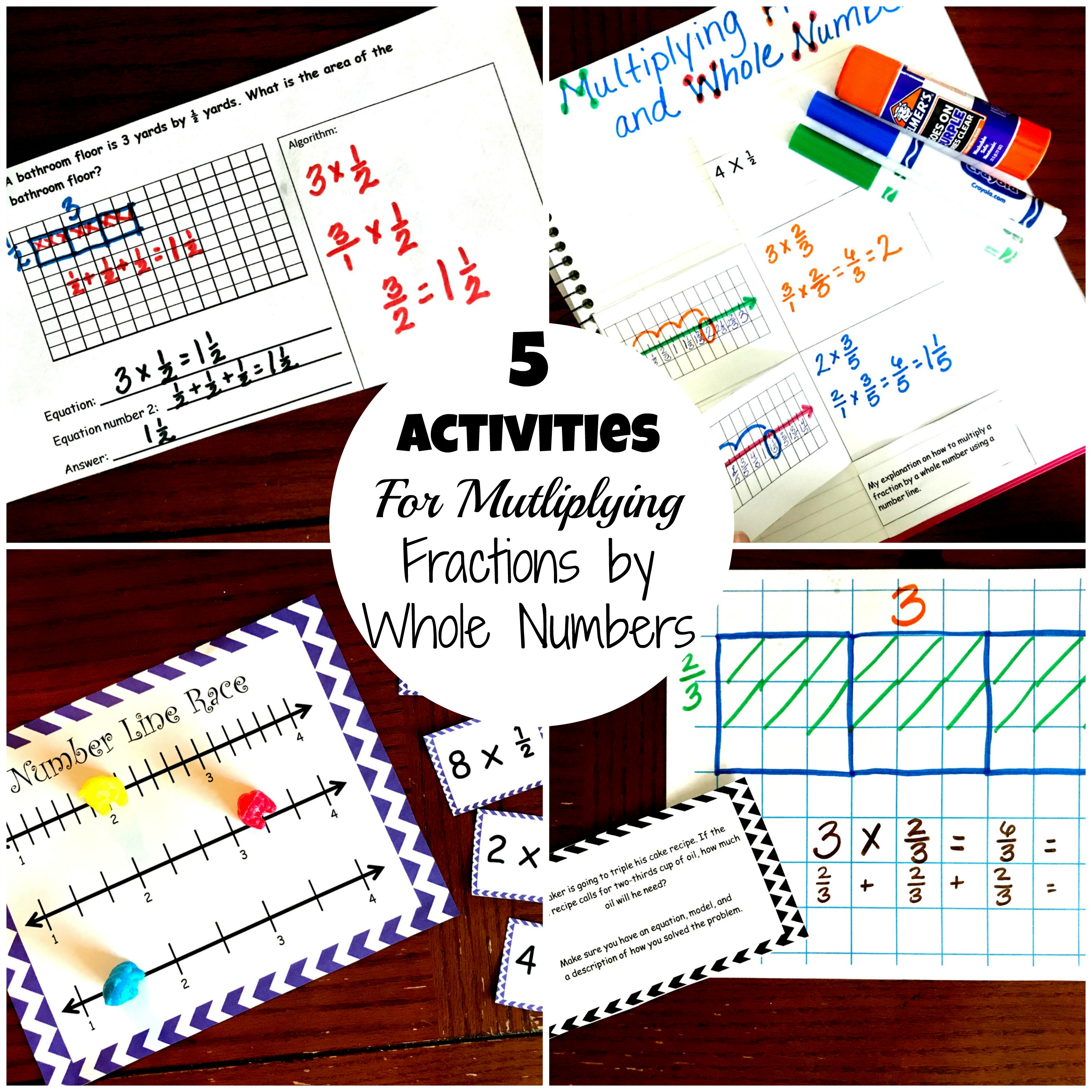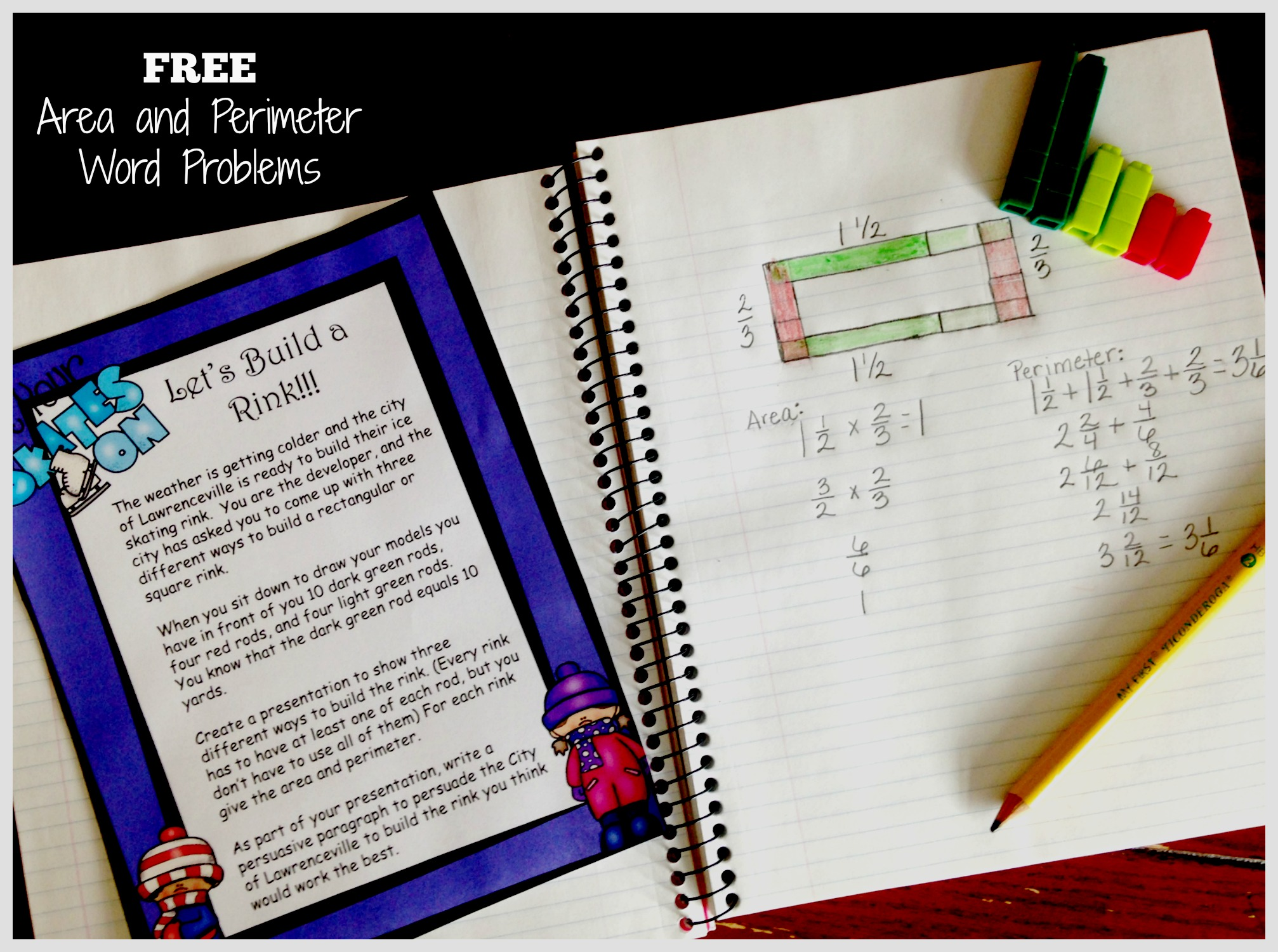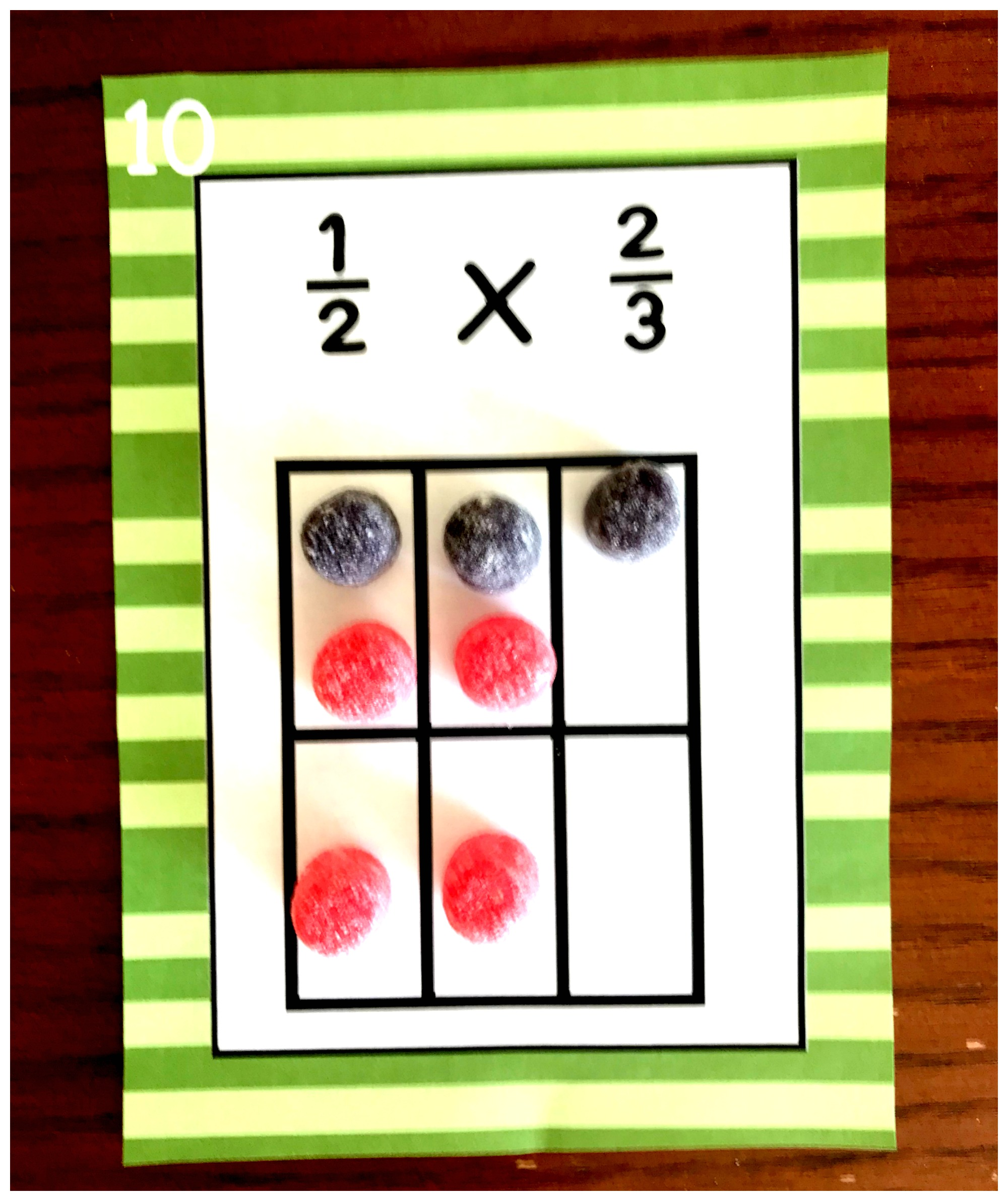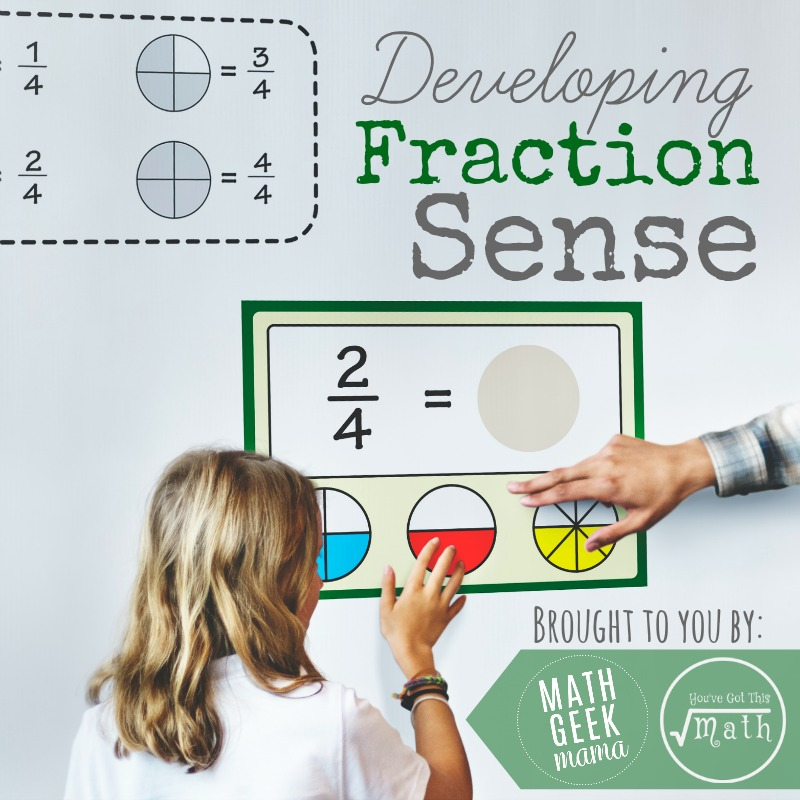Home » Math » One Free, Hands – On Multiplying Fractions Activity

One Free, Hands – On Multiplying Fractions Activity

This free multiplying fractions activity is a great way for children to use visual models or arrays to help them multiply a fraction by a fraction.The free printable can be found at the END of this post. Click on the blue “get your free printable here” button.

We are a participant in the Amazon Services LLC Associates Program, an affiliate advertising program designed to provide a means for us to earn fees by linking to Amazon.com and affiliated sites.

This post may also contain affiliate links to other bloggers too. There is no extra cost to you, but if you choose to purchase, I receive a small percentage.

It’s my passion! Helping children to understand math. Not just be able to answer a math question by plugging in numbers to a formula, but to understand why that formula works.

Today’s Multiplying Fractions Activity is a way to not just teach children how to multiply a fraction by a fraction, but to show them why and how it works.

Need to start with whole numbers by a fraction? This may help.or jump up to mixed numbers by mixed numbers with this activityWhat You Need!

This is such a simple activity to do, and yet it is a powerful way to help children actually see what is happening when you multiply fractions by fractions.

1. First, print off the cards on card stock paper, and the recording sheet on regular paper.
2. Next, cut out and laminate the cards.
3. Gather up Gum Drops and pencils and you are ready to go.

Step by Steps For Using this Multiplying Fraction Activity• To begin, the children look at the first fraction. They gather up one color of Gum Drops (in the example above they used purple).

• Next, they figure out they can fill in 1/2 of the array using that color of Gum Drops. With the array that has already been drawn for them, they can not fill in 1/2 going vertical, up and down. But if you look at the array horizontally, you can see that it has been divided into two equal parts. Since the numerator is one, our students should fill in one horizontal row with one color of Gum Drops.

• Now it is time to take a look at the second fraction, 2/3. Since we have already filled in the horizontal part of the array, it is time to fill in the vertical part which is already divided up into three equal parts. Our numerator is two, so two of those parts need to filled in with a different color Gum Drop. In the example above, that is red Gum Drops.

Finally, it is answer time. To figure out the answer we look at the whole array. The first question to ask our kiddos is, “How many parts are there in the whole array?”

• Hopefully, you get the answer six….and guess what? That is the denominator.

Next, ask them how many of the parts have two Gum Drops.

• This time you should get the answer 2…..and that is the numerator.

The answer is 2/3….and your children have solved a fraction by fraction problem.

Next Steps

After your students are understanding how the modeling works, you could take the next step and introduce how to multiply a fraction by a fraction without drawing an array. And of course, I love doing connecting the two through questions!

1. How do we get the denominator? Can we figure out the denominator without counting each box? (The goal here is to help them see that we can find the denominator by figuring out the area of the array…..length x width.
2. How did we get the numerator? Can we figure that out without counting each box? (Again the goal is to help them see that you multiplied the length time the width of the area that has two Gum Drops in it)

Now all you have to do is show them that when multiplying a fraction by a fraction you…..

1. Multiply the numerators
2. Multiply the denominators
3. Simplify if necessary.

I hope you enjoy this Multiplying Fraction Activity that helps children truly visualize this concept.

You’ve Got This

Rachel

You May Also Like:

This is a series that Math Geek Mama and I did together. It walks you through numerous ideas on helping children visualize fractions…not just memorize how to solve a problem.